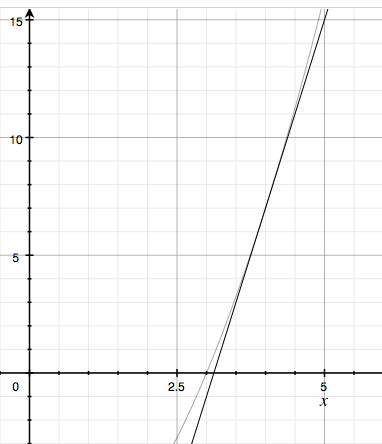# Find the slope of the line tangent to f(x)= x^2 - 9 at x= 4 and plot the point of tangency.

## Question:

Find the slope of the line tangent to {eq}f(x)= x^2 - 9 {/eq} at x= 4 and plot the point of tangency.

## Tangent Line

We can construct the equation of any line as long as we have both a point and a slope. For a tangent line, we can find both of these pieces of information by examining a function at a single point. This is because the point comes from the function itself and the slope from its derivative.

Let's find the tangent line to this function at the given point. To do so, we will need to know two things: the value of this function at this point and the value of the derivative to this function at this point.

{eq}\begin{align*} &f(x) = x^2-9 && f(4) = (4)^2 - 9 = 16-9 = 7\\ &f'(x) = 2x && f'(4) = 2(4) = 8 \end{align*} {/eq}

We can use point slope form to find this tangent line by using the value of the derivative (8) as the slope and (4, 7) as the point.

{eq}y-7 = 8(x-4)\\ y = 8x -25 {/eq}

Both the original function and the tangent line is graphed below. Note that the line touches the function with the same instantaneous slope at that point.